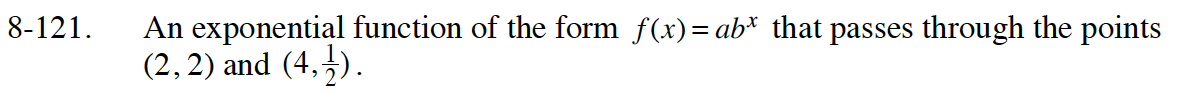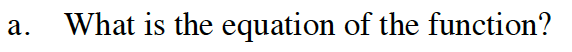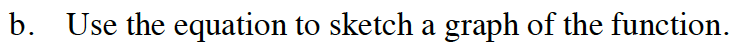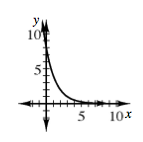### Home > INT1 > Chapter Ch8 > Lesson 8.2.3 > Problem8-121

8-121.
1. An exponential function of the form f(x) = abx that passes through the points (2, 2) and (4,). 8-121 HW eTool (Desmos). Homework Help ✎

1. What is the equation of the function?

2. Use the equation to sketch a graph of the function.Read Math Notes box in Lesson 8.2.2 on curve fitting an exponential function.

Create two equations using the points (2,2) and (4,1/2).
Equation 1: 2 = ab²
Equation 2: 1/2 = ab4

Isolate a for both equations. Use the Equal Values Method to solve for b.

Substitute b into one of the original equations, and solve for a.Use the eTool below to help solve the problem.
Click the link at the right to view full version of the eTool: Int1 8-121 HW eTool.